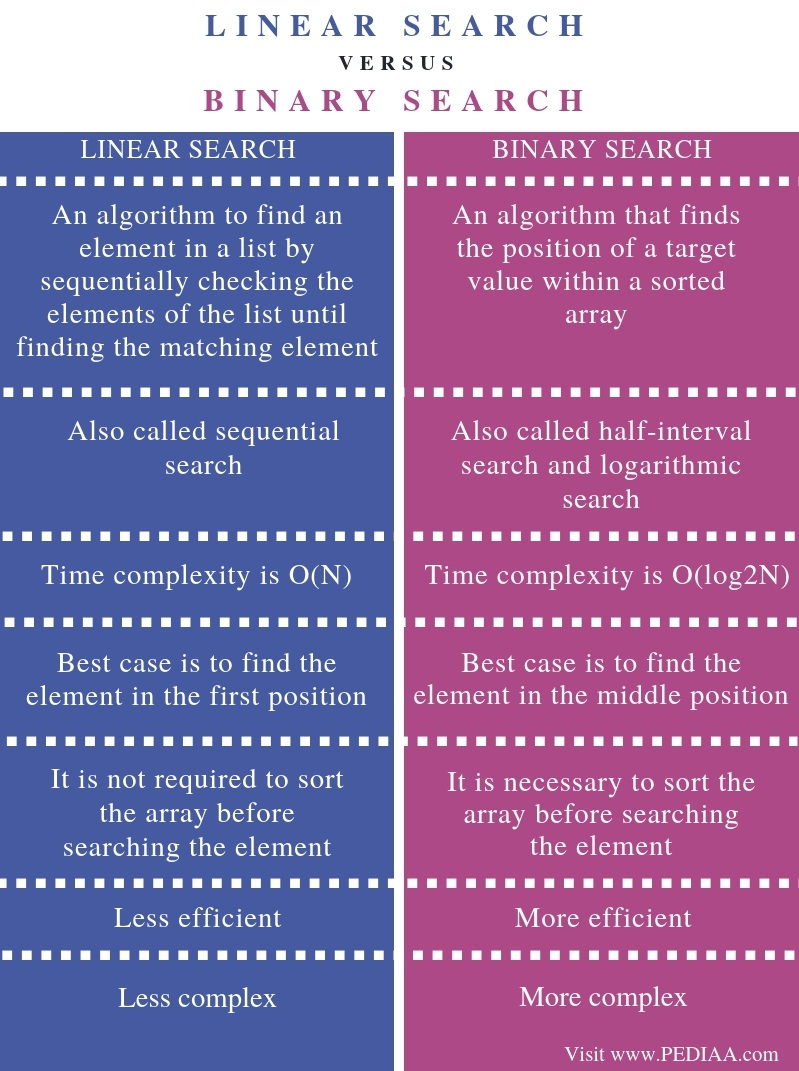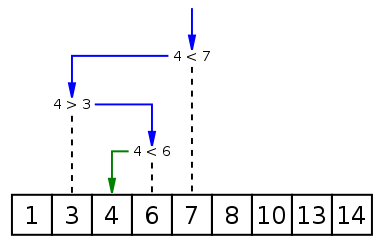What is the Difference Between Linear Search and Binary Search

The main difference between linear search and binary search is that a binary search (also known as a half-interval search or logarithmic search) is more efficient and takes minimum time to search an element than a linear search (or sequential search).

Searching is an operation that allows finding an element in a particular data structure such as an array. There are two search types as linear search and binary search. Linear search checks the elements of an array one by one in a sequential order to find whether the required item is present in the array. On the other hand, binary search is a more efficient algorithm than linear search as it searches the item by comparing it with the middle element.

Key Areas Covered

1. What is Linear Search
– Definition, Functionality
2. What is Binary Search
– Definition, Functionality
3. What is the Difference Between Linear Search and Binary Search
– Comparison of Key Differences

Key Terms

Binary search, Linear Search, Searching AlgorithmLinear search is a simple searching algorithm. Here, the searching occurs from one item after the other. That is; this algorithm checks every item and checks for a matching item of that. If the item is not present, searching continues until the end of the data. Therefore, linear search is an algorithm that allows going through each element in an array to locate the given item.

In a linear search, the time consumption or the number of comparisons to search an element helps to determine the efficiency of the algorithm. If the element we search is in the first position of the data structure, it requires only one comparison. When the required element is in the last position, it requires an N number of comparisons to find the element. Here, the N refers to the number of data items.

Binary search is a fast algorithm. But, it is necessary to sort the data items before performing a binary search. It finds the item by comparing the middle most item of the collection. Hence, the binary search takes less time to search a given item with less number of comparisons as it involves finding the middle element and comparing the middle element with the element to search.In a binary search, if the middle element is the required element, that index returns. If the middle item is higher than the searched item, then the searched item is in the left subarray of the middle item. Otherwise, the items are in the right subarray of the middle item. And, this process continues on the subarray until the subarray size becomes zero. In this algorithm, the number of items to search reduces each time.

Definition

Linear search is an algorithm to find an element in a list by sequentially checking the elements of the list until finding the matching element. Binary search is an algorithm that finds the position of a target value within a sorted array. Thus, this is the main difference between linear search and binary search.

Synonyms

Sequential search is another term for linear search whereas half-interval search or logarithmic search refers to the same binary search.

Time complexity

The time complexity of a linear search is O(N) while the time complexity of a binary search is O(log2N). Hence, this is another difference between linear search and binary search.

Best Case

Furthermore, the best case in a linear search is to find the element in the first position while the best case in binary search is to find the element in the middle position.

Sorting the Array

In a linear search, it is not required to sort the array before searching the element. However, in a binary search, it is necessary to sort the array before searching the element. Therefore, the prerequisite to sort the array makes a difference between linear search and binary search.

Efficiency

One other difference between linear search and binary search is their efficiency. Binary search is more efficient than linear search.

Simplicity

Besides, binary search is more complex than linear search.

Conclusion

Linear search and binary search are two algorithms to search an element in a data structure such as an array. Binary search is efficient and fast than linear search, but it is mandatory to sort the array first before performing the search operation. Thus, the main difference between linear search and binary search is that binary search is more efficient and takes minimum time to search an element, when compared to linear search.

Reference:

1. “Linear Search.” Wikipedia, Wikimedia Foundation, 13 Dec. Available here.
2. “Binary Search Algorithm.” Wikipedia, Wikimedia Foundation, 26 Dec. 2018, Available here.

Image Courtesy:

1. “Binary search into array” By Tushe2000 – Template:LoStrangolatore (Public Domain) via Commons Wikimedia